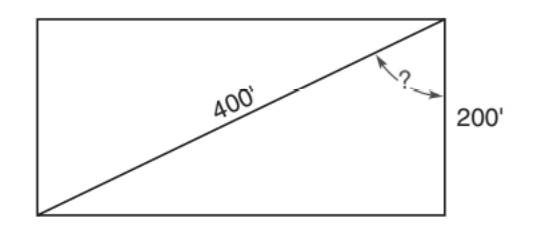Chapter 5.5, Problem 25EElementary Geometry For College St...

7th Edition
Alexander + 2 others
ISBN: 9781337614085

Solutions

Chapter
SectionElementary Geometry For College St...

7th Edition
Alexander + 2 others
ISBN: 9781337614085
Textbook Problem

A jogger runs along two sides of an open rectangular lot. If the first side of the lot is 200 ft long and the diagonal distance across the lot is 400 ft, what is the measure of the angle formed by the 200 -ft and 400 -ft dimensions? To the nearest foot, how much farther does the jogger run by travelling the two sides of the block rather than the diagonal distance across the lot?To determine

To find:

The measure of the angle formed by the 200 ft. and 400 ft. dimensions and the distance by which the jogger ran farther by travelling the two sides of the block rather than the diagonal across the lot.

Explanation

Approach:

For a right triangle, for which the measure of the interior angles 30°, 60°, and 90°; if ‘a’ is the length of measure of the shorter leg; opposite to the angle 30°, then the length of the other two sides is given by

Length of the longer leg (opposite to 60°) =a3

Length of the hypotenuse (opposite to 90°)=2a.

In general

Length of the longer leg =3× (Length of the shorter leg)

Length of the hypotenuse =2× (Length of the shorter leg)

Calculation:

Given,

Jogger runs along two sides of an open rectangular lot, the first side of the lot is 200 ft. long and the diagonal across the lot is 400 ft.

The width of the rectangular lot =200 ft.

The diagonal of the rectangular lot =400 ft.

Let the rectangular lot be named as ABCD with AC as diagonal.

Now, the triangle ABC formed by the diagonal AC of the rectangle is a right triangle with

Right angle at B,

AC=400 ft = Hypotenuse

BC=200 ft.

Here, we find that in ABC

AC=2BC

Hypotenuse =2(BC)

But, by the 30-60-90 theorem of a triangle,

30°-60°-90° theorem.

In a right triangle whose angle measure 30°, 60°, and 90°, the hypotenuse has a length equal to twice the length of the shorter leg, and the longer leg is the product of 3 and the length of the shorter leg.

Hypotenuse =2× (Length of the shorter side)

Thus, BC is the shorter side of the right triangle which always lies opposite to 30°.

Hence,

mA=30°

Further, AB should be the longer side of the right , which should lie opposite to the angle 60°

In right ABC apply phythagorean theorem to find AB the second side of the rectangular lot

Still sussing out bartleby?

Check out a sample textbook solution.

See a sample solution

The Solution to Your Study Problems

Bartleby provides explanations to thousands of textbook problems written by our experts, many with advanced degrees!

Get Started

Find the limit: limx3x2+x12x3

Calculus: An Applied Approach (MindTap Course List)

Solve the equations in Exercises 112 for x (mentally, if possible). x+1=2x+2

Finite Mathematics and Applied Calculus (MindTap Course List)

Evaluate the integral. 28. tan2xsec3xdx

Single Variable Calculus: Early Transcendentals

In Exercises 116, determine whether the argument is valid. pqqrpr

Finite Mathematics for the Managerial, Life, and Social Sciences